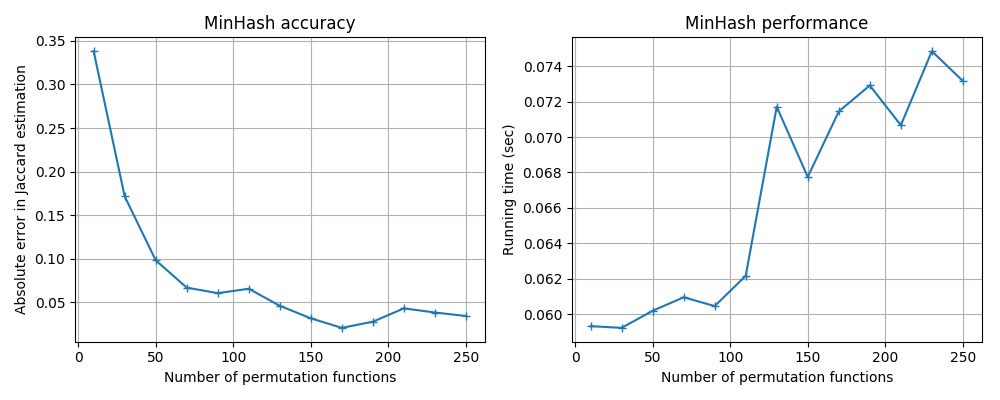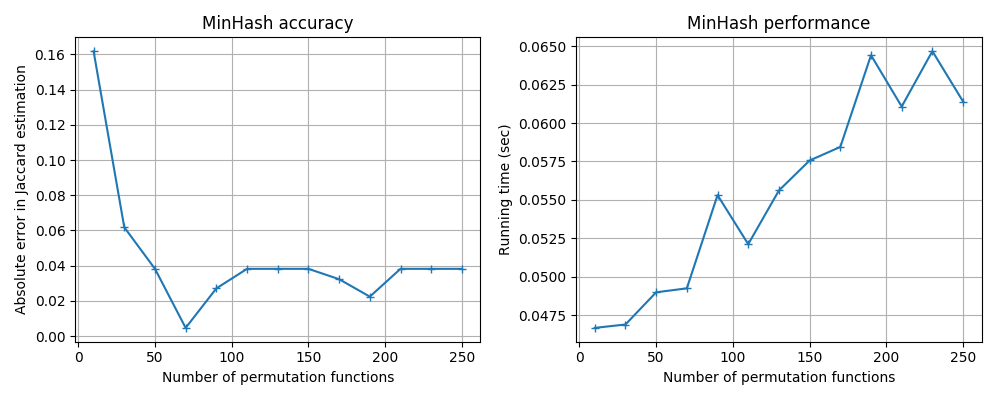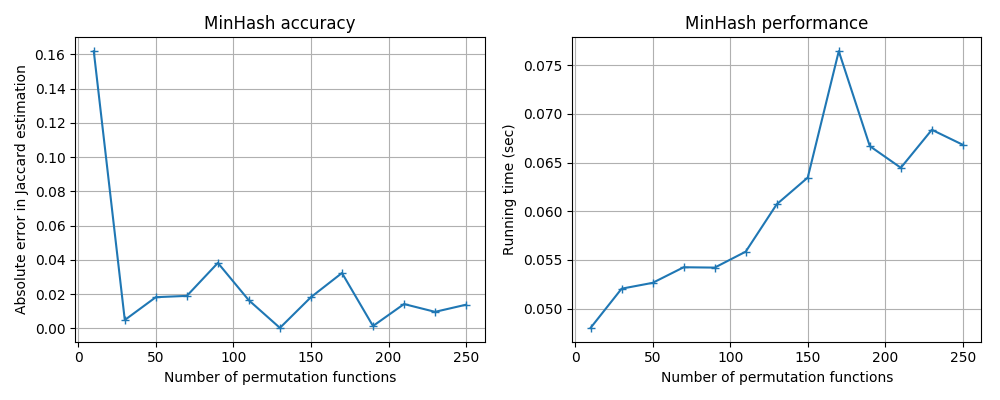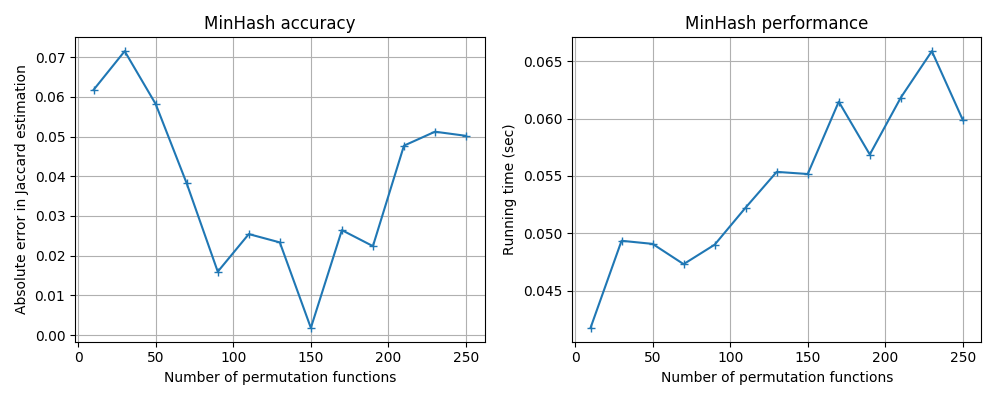# MinHash¶

datasketch.MinHash lets you estimate the Jaccard similarity (resemblance) between sets of arbitrary sizes in linear time using a small and fixed memory space. It can also be used to compute Jaccard similarity between data streams. MinHash is introduced by Andrei Z. Broder in this paper

from datasketch import MinHash

data1 = ['minhash', 'is', 'a', 'probabilistic', 'data', 'structure', 'for',
'estimating', 'the', 'similarity', 'between', 'datasets']
data2 = ['minhash', 'is', 'a', 'probability', 'data', 'structure', 'for',
'estimating', 'the', 'similarity', 'between', 'documents']

m1, m2 = MinHash(), MinHash()
for d in data1:
m1.update(d.encode('utf8'))
for d in data2:
m2.update(d.encode('utf8'))
print("Estimated Jaccard for data1 and data2 is", m1.jaccard(m2))

s1 = set(data1)
s2 = set(data2)
actual_jaccard = float(len(s1.intersection(s2)))/float(len(s1.union(s2)))
print("Actual Jaccard for data1 and data2 is", actual_jaccard)


You can adjust the accuracy by customizing the number of permutation functions used in MinHash.

# This will give better accuracy than the default setting (128).
m = MinHash(num_perm=256)


The trade-off for better accuracy is slower speed and higher memory usage. Because using more permutation functions means

1. more CPU instructions for every data value hashed and

2. more hash values to be stored.

The speed and memory usage of MinHash are both linearly proportional to the number of permutation functions used.You can union two MinHash object using the merge function. This makes MinHash useful in parallel MapReduce style data analysis.

# The makes m1 the union of m2 and the original m1.
m1.merge(m2)


MinHash can be used for estimating the number of distinct elements, or cardinality. The analysis is presented in Cohen 1994.

# Returns the estimation of the cardinality of
# all data values seen so far.
m.count()


If you are handling billions of MinHash objects, consider using datasketch.LeanMinHash to reduce your memory footprint.

## Use Different Hash Functions¶

MinHash by default uses the SHA1 hash funciton from Python’s built-in hashlib library. You can also change the hash function using the hashfunc parameter in the constructor.

# Let's use MurmurHash3.
import mmh3

# We need to define a new hash function that outputs an integer that
# can be encoded in 32 bits.
def _hash_func(d):
return mmh3.hash32(d)

# Use this function in MinHash constructor.
m = MinHash(hashfunc=_hash_func)


Different hash functions have different performance-accuracy trade-off, you can use the benchmark code in benchmark/minhash_benchmark.py to run some tests. Here are the results for some popular hash functions available in Python.

MurmurHash3: mmh3xxhashFarmhash